# Cone side

Calculate the volume and area of the cone whose height is 10 cm and the axial section of the cone has an angle of 30 degrees between height and the cone side.

Result

V =  349.066 cm3
S =  314.159 cm2

#### Solution:

$h = 10 \ cm \ \\ A = 30 ^\circ \rightarrow rad = 30 ^\circ \cdot \ \dfrac{ \pi }{ 180 } \ = 30 ^\circ \cdot \ \dfrac{ 3.1415926 }{ 180 } \ = 0.5236 \ = π/6 \ \\ \ \\ \tan A = r:h \ \\ \ \\ r = h \cdot \ \tan(A) = 10 \cdot \ \tan(0.5236) \doteq 5.7735 \ cm \ \\ \ \\ S_{ 1 } = \pi \cdot \ r^2 = 3.1416 \cdot \ 5.7735^2 \doteq 104.7198 \ cm^2 \ \\ \ \\ V = \dfrac{ 1 }{ 3 } \cdot \ S_{ 1 } \cdot \ h = \dfrac{ 1 }{ 3 } \cdot \ 104.7198 \cdot \ 10 \doteq 349.0659 = 349.066 \ cm^3$
$s = \sqrt{ h^2+r^2 } = \sqrt{ 10^2+5.7735^2 } \doteq 11.547 \ cm \ \\ S_{ 2 } = \pi \cdot \ r \cdot \ s = 3.1416 \cdot \ 5.7735 \cdot \ 11.547 \doteq 209.4395 \ cm^2 \ \\ S = S_{ 1 }+S_{ 2 } = 104.7198+209.4395 \doteq 314.1593 = 314.159 \ cm^2$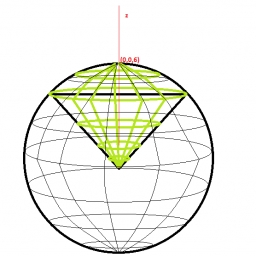Our examples were largely sent or created by pupils and students themselves. Therefore, we would be pleased if you could send us any errors you found, spelling mistakes, or rephasing the example. Thank you!

Leave us a comment of this math problem and its solution (i.e. if it is still somewhat unclear...):Be the first to comment!Tips to related online calculators
Check out our ratio calculator.
Tip: Our volume units converter will help you with the conversion of volume units.
Pythagorean theorem is the base for the right triangle calculator.

## Next similar math problems:

1. One side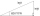One side is 36 long with a 15° incline. What is the height at the end of that side?
2. Right angled triangle 3Side b = 1.5, hypotenuse angle A = 70 degrees, Angle B = 20 degrees. Find its unknown sides length.
3. Right triangleCalculate the length of the remaining two sides and the angles in the rectangular triangle ABC if a = 10 cm, angle alpha = 18°40'.
4. Tetrahedral pyramidDetermine the surface of a regular tetrahedral pyramid when its volume is V = 120 and the angle of the sidewall with the base plane is α = 42° 30´.
5. Trapezoid MOThe rectangular trapezoid ABCD with right angle at point B, |AC| = 12, |CD| = 8, diagonals are perpendicular to each other. Calculate the perimeter and area of ​​the trapezoid.
6. Diagonals of pentagonCalculate the diagonal length of the regular pentagon: a) inscribed in a circle of radius 12dm; b) a circumscribed circle with a radius of 12dm.
7. Church tower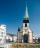Archdeacon church in Usti nad Labem has diverted tower by 186 cm. The tower is 65 m high. Calculate the angle by which the tower is tilted. Result write in degree's minutes.
8. The aspect ratioThe aspect ratio of the rectangular triangle is 13: 12: 5. Calculate the internal angles of the triangle.
9. Ratio iso triangleThe ratio of the sides of an isosceles triangle is 7:6:7 Find the base angle to the nearest answer correct to 3 significant figure.
10. Isosceles triangle 10In an isosceles triangle, the equal sides are 2/3 of the length of the base. Determine the measure of the base angles.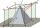The height of the regular quadrangular pyramid is 6 cm, the length of the base is 4 cm. What is the angle between the ABV and BCV planes?Approximately at what height is the cloud we see under an angle of 26°10' and see the Sun at an angle of 29°15' and the shade of the cloud is 92 meters away from us?I have coordinates of square vertices A / -3; 1/and B/1; 4 /. Find coordinates of vertices C and D, C 'and D'. Thanks Peter.Two forces with magnitudes of 25 and 30 pounds act on an object at angles of 10° and 100° respectively. Find the direction and magnitude of the resultant force. Round to two decimal places in all intermediate steps and in your final answer.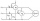Two wattmeters are connected to measuring power in a 3 phase balanced load. Determine the total power and power factor if the two wattmeters read 1000 watts each (1) both positive and (2) second reading is negativeThe angle of a straight road is approximately 12 degrees. Determine the percentage of this road.Between points A and B is 50m. From A we see a tree at an angle 18°. From point B we see the tree in three times bigger angle. How tall is a tree?